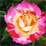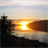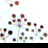# 'If...Then' Code for Field Calculator

1877
4
11-14-2011 10:37 AMNew Contributor
I completed a Model that overlays several polygon layers, clips out a specified section, dissolves according to certain attributes and sums the acreage.  What I want to do is add another Calculate Field entry into my Model, but I can't get the Field Calculation correct.  The Fields I want to select from are [CAUVLCType] and [MUSYM].  The fields are coded-value domains and descriptions.  What I've tried is similar to Label expressions:

Function
if ([CAUVLCType] = 'CROP' AND [MUSYM] = 'NpA') then
[Soil_Acreage] * 460
elseif ([CAUVLCType] = 'WOOD' AND [MUSYM] = 'NpA') then
[Soil_Acreage] * 100
End if
End Function

Can someone provide me a couple lines of code that will work on a copy/paste basis which I can repeat and enter different field descriptions as needed?!

Thanks, Jeff
Tags (3)
4 RepliesbyMVP Honored Contributor
I completed a Model that overlays several polygon layers, clips out a specified section, dissolves according to certain attributes and sums the acreage.  What I want to do is add another Calculate Field entry into my Model, but I can't get the Field Calculation correct.  The Fields I want to select from are [CAUVLCType] and [MUSYM].  The fields are coded-value domains and descriptions.  What I've tried is similar to Label expressions:

Function
if ([CAUVLCType] = 'CROP' AND [MUSYM] = 'NpA') then
[Soil_Acreage] * 460
elseif ([CAUVLCType] = 'WOOD' AND [MUSYM] = 'NpA') then
[Soil_Acreage] * 100
End if
End Function

Can someone provide me a couple lines of code that will work on a copy/paste basis which I can repeat and enter different field descriptions as needed?!

Thanks, Jeff

Drop the Function brackets.  They are not used in the Field Calculator.  Also, if you are using VB Script, use double quotes not single quotes for literal strings.  Finally you have to return a variable value to the expression in order to output your calculated values.  Try these settings (use the expression builder in the Field Calculator tool):

Parser:  VB Script

Code Block:
Dim Output
If ([CAUVLCType] = "CROP" AND [MUSYM] = "NpA") then
Output = [Soil_Acreage] * 460
elseif ([CAUVLCType] = "WOOD" AND [MUSYM] = "NpA") then
Output = [Soil_Acreage] * 100
End if

Expression:  OutputNew Contributor

Dim [Dense95]
IF [JobDensity] >= [Mean] + [SD] * [z164] THEN
[Dense95] = 1
ELSE
[Dense95] =  0
end IF

What am I doing wrong? [Dense95] is the field where I want the output to auto populate.

lesterbyEsri Regular Contributor
Try declaring and using teh variable as:

```Dim Dense95
```

Without the []s.Occasional Contributor III
You cannot calculate the field directly in the code block.
You can reference a field, as in your If statement,
But you cannot change a field, even if it is the one you are calculating.

The code block acts like a function (even though it doesn't use Function tags), and has to return the value it is calculating to the calculation expression. That is why the code block is called Pre-Logic Script Code in the Field calculator: it is executed BEFORE the field is calculated.

As in rfairhur24's example, make the codeblok output a variable:
Output = [Soil_Acreage] * 460

Then put the variable (in thios case, Output) in the expression (in the Field Calculator, it is the box below the code block box. The one headed: Soil_Acreage =

In the python code, the output variable goes in the expression parameter
CalculateField_management (in_table, field, expression, {expression_type}, {code_block})
While the code block (that sets the variable to the value you need) is in the code_block variable.